Dividend Decision (Part - 2) - Accountancy and Financial Management

# Dividend Decision (Part - 2) - Accountancy and Financial Management Notes | Study Accountancy and Financial Management - B Com

## Document Description: Dividend Decision (Part - 2) - Accountancy and Financial Management for B Com 2022 is part of Accountancy and Financial Management preparation. The notes and questions for Dividend Decision (Part - 2) - Accountancy and Financial Management have been prepared according to the B Com exam syllabus. Information about Dividend Decision (Part - 2) - Accountancy and Financial Management covers topics like and Dividend Decision (Part - 2) - Accountancy and Financial Management Example, for B Com 2022 Exam. Find important definitions, questions, notes, meanings, examples, exercises and tests below for Dividend Decision (Part - 2) - Accountancy and Financial Management.

Introduction of Dividend Decision (Part - 2) - Accountancy and Financial Management in English is available as part of our Accountancy and Financial Management for B Com & Dividend Decision (Part - 2) - Accountancy and Financial Management in Hindi for Accountancy and Financial Management course. Download more important topics related with notes, lectures and mock test series for B Com Exam by signing up for free. B Com: Dividend Decision (Part - 2) - Accountancy and Financial Management Notes | Study Accountancy and Financial Management - B Com
 1 Crore+ students have signed up on EduRev. Have you?

Relevance of Dividend
According to this concept, dividend policy is considered to affect the value of the firm. Dividend relevance implies that shareholders prefer current dividend and there is no direct relationship between dividend policy and value of the firm. Relevance of dividend concept is supported by two eminent persons like Walter and Gordon.

Walter’s Model
Prof. James E. Walter argues that the dividend policy almost always affects the value of the firm.
Walter model is based in the relationship between the following important factors:

• Rate of return I
• Cost of capital (k)

According to the Walter’s model, if r > k, the firm is able to earn more than what the shareholders could by reinvesting, if the earnings are paid to them. The implication of r >k is that the shareholders can earn a higher return by investing elsewhere. If the firm has r = k, it is a matter of indifferent whether earnings are retained or distributed.

Assumptions
Walters model is based on the following important assumptions:

1. The firm uses only internal finance.
2. The firm does not use debt or equity finance.
3. The firm has constant return and cost of capital.
4. The firm has 100 recent payout.
5. The firm has constant EPS and dividend.
6. The firm has a very long life.

Walter has evolved a mathematical formula for determining the value of market share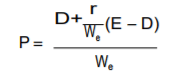Where,
P = Market price of an equity share
D = Dividend per share
r = Internal rate of return
E = Earning per share
Ke = Cost of equity capital

Example 5.
From the following information supplied to you, ascertain whether the firm is following an optional dividend policy as per Walter’s Model?

 Total Earnings Rs. 2,00,000 No. of equity shares (of Rs. 100 each ) 20,000 Dividend paid Rs. 1,00,000 P/E Ratio 10 Return Investment 15%

The firm is expected to maintain its rate on return on fresh investments. Also find out what should be the E/P ratio at which the dividend policy will have no effect on the value of the share? Will your decision change if the P/E ratio is 7.25 and interest of 10 %?
Solution.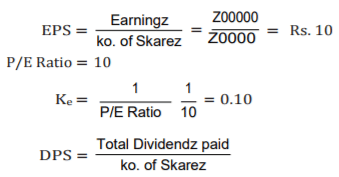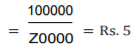The value of the share as per Walter’s Model is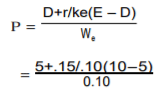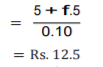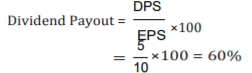r >Ke therefore by distributing 60% of earnings, the firm is not following an optional dividend policy. In this case, the optional dividend policy for the firm would be to pay zero dividend and the Market Price would be: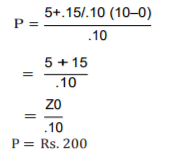So, the MP of the share can be increased by following a zero payout, of the P/E is 7.25 instead of 10 then the Ke =1=0.138 and in this case Ke>r and the MP of the share is 7.25.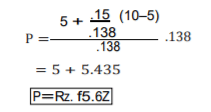Example 6.
The earnings per share of a company are Rs. 80 and the rate of capitalization applicable to the company is 12%. The company has before it an option of adopting a payment ratio of 25% (or) 50%(or) 75%. Using Walter’s formula of dividend payout, compute the market value of the company’s share of the productivity of retained earnings (i) 12% (ii) 8% (iii) 5%.
Solution.
on E = 10 and Ke=12%=0.12
As per Walter’s Model, the market price of a share is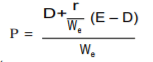(A) If payout ratio is 25%
(i) r=12%=0.12, D=25%of 10=Rs. 2.50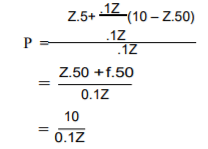= Rs. 83.33
R = 8% = 0.08
R = 8% = 0.08, D = 25% of 10 = Rs. 2.50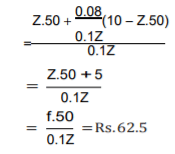Example 7.
From the following data, calculate the MP of a share of ABC Ltd., under (i) Walter’s formula; and (ii) Dividend growth model.
EPS = Rs. 10   DPS = Rs. 6
Ke = 18%          r =25%
retention ratio (b) = 45%
Solution.
(i) Walter’s Model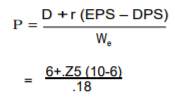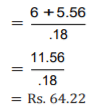(ii) Dividend Growth Model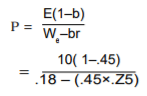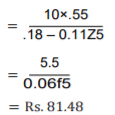Criticism of Walter’s Model
The following are some of the important criticisms against Walter model: Walter model assumes that there is no extracted finance used by the firm. It is not practically applicable. There is no possibility of constant return. Return may increase or decrease, depending upon the business situation. Hence, it is applicable. According to Walter model, it is based on constant cost of capital. But it is not applicable in the real life of the business.

Gordon’s Mode
Myron Gorden suggest one of the popular model which assume that dividend policy of a firm affects its value, and it is based on the following important assumptions:

1. The firm is an all equity firm.
2. The firm has no external finance.
3. Cost of capital and return are constant.
4. The firm has perpectual life.
5. There are no taxes.
6. Constant relation ratio (g=br).
7. Cost of capital is greater than growth rate (Ke >br).

Gordon’s model can be proved with the help of the following formula: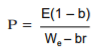Where,
P = Price of a share
E = Earnings per share
1 – b = D/p ratio (i.e., percentage of earnings distributed as dividends)
Ke = Capitalization rate
br = Growth rate = rate of return on investment of an all equity firm.

Example 8.
Raja company earns a rate of 12% on its total investment of Rs. 6,00,000 in assets. It has 6,00,000 outstanding common shares at Rs. 10 per share. Discount rate of the firm is 10% and it has a policy of retaining 40% of the earnings. Determine the price of its share using Gordon’s Model. What shall happen to the price of the share if the company has payout of 60% (or) 20%?
Solution.
According to Gordon’s Model, the price of a share is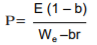Given: E = 12% of Rs.10=Rs. 1.20
r = 12%=0.12
K =10%=0.10
t = 10%=0.10
b = 40%=0.40
Put the values in formula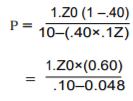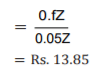If the firm follows a policy of 60% payout then b=20% =0.20
The price is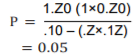r=4% =0.04, D =25% of 10=2.50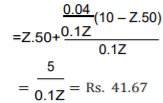If payout ratio is 50%,D=50% of 10=Rs. 5
r=12%=0.12, D=50% of 10 = Rs. 5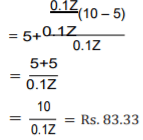r = 8% = 0.08, D = 50% of 10 = 5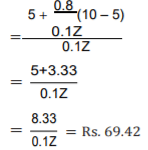r = 4% = 0.04, D = 50% of 10 = 5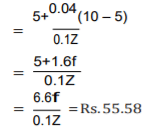C. If payout ratio is 75%
D = 75% of 10 = 7.50
(i) r = 12% = 0.12, D = 75% of 10 = 7.50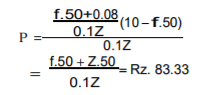(ii) r = 8% = 0.8, D = 75% of 10 = 7.50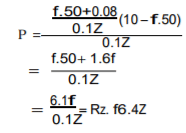(iii) r = 4% = 0.04, D = 75% of 10 = 7.50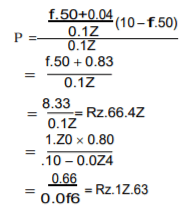If the payout is 20% the value of b=0.60 and the price of the share is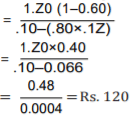Criticism of Gordon’s Model
Gordon’s model consists of the following important criticisms: Gordon model assumes that there is no debt and equity finance used by the firm. It is not applicable to present day business. Ke and r cannot be constant in the real practice. According to Gordon’s model, there are no tax paid by the firm. It is not practically applicable.

Limitation on Dividend Payments:
The firm has the following limitations in paying dividends.
The management of the firm while making decision in paying out dividends to its shareholders should also analyse these problems:

i. Cash Requirements:
Many firms are unable to pay dividends regularly. A company which is going through its gestation period or is small in nature and is trying to expand its business has the problem of paying high dividends.
If it does, it will be surrounded by inefficiency because of the insufficiency of cash. Sometimes, a firm has the problem of tying up all resources in inventories or in the commitment of purchasing long-term investments. This acts as a restraint of the firm to pay out dividends.

ii. Limitations Placed by Creditors:
Sometimes, a firm requires funds for long-term purpose and to fulfil this obligation it makes, the use of funds on long-term loans. While taking these loans the firm makes an arrangement with the creditors that it will not pay dividends to its shareholders till its debt equity ratio depicts 2:1.
Sometimes, the firm also makes contractual obligations with its creditors to maintain a certain pay-out ratio till the time that it is using the loan facilities. Under these contractual obligations, the firm cannot pay more than the dividends it can, or is allowed to pay, under the agreement.

iii. Legal Constraints:
In India, there are many legal constraints in payment of dividends. The payment of dividends is subject to government policy and tax laws. This restraint also covers bonds, debentures and equity shares.
There are regulatory authorities such as Reserve Bank of India, Securities Exchange Board of India, Insurance Regulatory Authority of India. Income Tax Act of India and Companies Act followed in India. These legal constraints should be carefully analysed before paying dividends to the shareholders.

The document Dividend Decision (Part - 2) - Accountancy and Financial Management Notes | Study Accountancy and Financial Management - B Com is a part of the B Com Course Accountancy and Financial Management.
All you need of B Com at this link: B Com

## Accountancy and Financial Management

35 videos|62 docs
 Use Code STAYHOME200 and get INR 200 additional OFF

## Accountancy and Financial Management

35 videos|62 docs

Track your progress, build streaks, highlight & save important lessons and more!

,

,

,

,

,

,

,

,

,

,

,

,

,

,

,

,

,

,

,

,

,

;